﻿ Weingarten变换下曲面的特征向量和特征值的一种求法

# Weingarten变换下曲面的特征向量和特征值的一种求法A Method for Finding Eigenvectors and Eigenvalues of Surfaces under Weingarten Transformation

Abstract: In this paper, by using the traditional method of solving the eigenvalues of matrix with order n in Advanced Algebra, the eigenvalues and corresponding eigenvectors of some regular surfaces under Weingarten transformation are obtained. Precisely, after obtaining all eigenvalues of characteristic equation of the Weingarten matrix, we solve the eigenvector corresponding to its eigenvalue. The biggest distinction is in that the eigenvector is expressed by the basis formed by tangent vectors of coordinate curves with respect to the tangent plane on given surface. Some examples of canonical surfaces are used to illustrate the methods for solving eigenvalues and eigenvector to their Weingarten transformations, which help us a better understanding of eigenvalue and eigenvector of surfaces.

1. 引言

2. 基本概念

n阶矩阵的特征值和特征向量和曲面上Weingarten变换下特征值和特征向量在求解的方法上有着许多相同之处，但它们本质上还是有很大的区别。大多数《高等代数》的教材对n阶矩阵的特征值和特征向量有如下定义：

$A\xi =\lambda \xi$ , (2.1)

$|A-\lambda I|={\lambda }^{n}-\left({a}_{11}+{a}_{22}+\cdot \cdot \cdot +{a}_{nn}\right){\lambda }^{n-1}+\cdot \cdot \cdot +{\left(-1\right)}^{n}|A|$

$I=\text{d}s=E\left(u,v\right)\text{d}{u}^{2}+2F\left(u,v\right)\text{d}u\text{d}v+G\left(u,v\right)\text{d}{v}^{2}$

$E\left(u,v\right)={r}_{u}\cdot {r}_{u}$ , $F\left(u,v\right)={r}_{u}\cdot {r}_{v}$ , $G\left(u,v\right)={r}_{v}\cdot {r}_{v}$ .

$II=-\text{d}r\cdot \text{d}n=L\left(u,v\right)\text{d}{u}^{2}+2M\left(u,v\right)\text{d}u\text{d}v+N\left(u,v\right)\text{d}{v}^{2}$

$L\left(u,v\right)={r}_{uu}\cdot n$ , $M\left(u,v\right)={r}_{uv}\cdot n$ , $N\left(u,v\right)={r}_{vv}\cdot n$ .

$\text{d}n={n}_{i}\text{d}{u}^{i}=-{\omega }_{i}^{j}\text{d}{u}^{i}{r}_{j}=-{\Omega }_{ik}{g}^{kj}{r}_{j}$

$\text{d}n=\left(\text{d}{u}^{1},\text{d}{u}^{2}\right)\left(\begin{array}{c}{n}_{1}\\ {n}_{2}\end{array}\right)=-\left(\text{d}{u}^{1},\text{d}{u}^{2}\right)\omega \left(\begin{array}{c}{r}_{1}\\ {r}_{2}\end{array}\right)=-\left(\text{d}{u}^{1},\text{d}{u}^{2}\right)\Omega {g}^{-1}\left(\begin{array}{c}{r}_{1}\\ {r}_{2}\end{array}\right)$ ,

$\omega =\Omega {g}^{-1}=\left(\begin{array}{cc}L& M\\ M& G\end{array}\right){\left(\begin{array}{cc}E& F\\ F& G\end{array}\right)}^{-1}=\left(\begin{array}{cc}\frac{LG-MF}{EG-{F}^{2}}& \frac{ME-LF}{EG-{F}^{2}}\\ \frac{MG-NF}{EG-{F}^{2}}& \frac{NE-MF}{EG-{F}^{2}}\end{array}\right)$ ,

$W:{T}_{p}↦{T}_{p}$ ,

$a={{a}^{i}{r}_{i}|}_{p}↦W\left(a\right)={a}^{i}W\left({{r}_{i}|}_{p}\right)$ ,

$W\left({{r}_{i}|}_{p}\right)={\left({\omega }_{i}^{j}{r}_{j}\right)|}_{p}$ ,

3. 关于Weingarten矩阵 $\omega$ 的性质

Weingarten矩阵的特征值方程为

${\lambda }^{2}-tr\omega \cdot \lambda +|\omega |=0$ ,

$\omega =\Omega {g}^{-1}=\left(\begin{array}{cc}\frac{LG-MF}{EG-{F}^{2}}& \frac{ME-LF}{EG-{F}^{2}}\\ \frac{MG-NF}{EG-{F}^{2}}& \frac{NE-MF}{EG-{F}^{2}}\end{array}\right)$ ,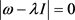知，

$tr\omega =tr\left(\Omega {g}^{-1}\right)=\frac{{g}_{11}{\Omega }_{22}-2{g}_{12}{\Omega }_{12}+{g}_{22}{\Omega }_{11}}{|g|}=\frac{EN-2FM+GL}{EG-{F}^{2}}$ ,

$|\omega |=|\Omega {g}^{-1}|=\frac{|\Omega |}{|g|}=\frac{LN-{M}^{2}}{EG-{F}^{2}}$ .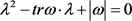.

$\begin{array}{c}\Delta =f={\left(tr\omega \right)}^{2}-4|\omega |\\ ={\left({\omega }_{1}^{1}+{\omega }_{2}^{2}\right)}^{2}-4\left({\omega }_{1}^{1}{\omega }_{2}^{2}-{\omega }_{1}^{2}{\omega }_{2}^{1}\right)\\ ={\left({\omega }_{1}^{1}-{\omega }_{2}^{2}\right)}^{2}+4{\omega }_{1}^{2}{\omega }_{2}^{1}\end{array}$

${\omega }_{1}^{1}=\frac{L}{E}$ , ${\omega }_{1}^{2}=\frac{M}{G}$ , ${\omega }_{2}^{1}=\frac{M}{E}$ , ${\omega }_{2}^{2}=\frac{N}{G}$ .

$f={\left({\omega }_{1}^{1}-{\omega }_{2}^{2}\right)}^{2}+4{\omega }_{1}^{2}{\omega }_{2}^{1}={\left(\frac{L}{E}-\frac{N}{G}\right)}^{2}+\frac{4{M}^{2}}{EG}\ge 0$ .

4. 求正则曲面特征值和特征向量的讨论

$r\left(u,v\right)=\left(u+v,u-v,uv\right)$ ，可得

${r}_{1}={r}_{u}=\left(1,1,v\right)$ , ${r}_{2}={r}_{v}=\left(1,-1,u\right)$ , ${r}_{1}×{r}_{2}={r}_{u}×{r}_{v}=\left(u+v,v-u,-2\right)$ ,

$n=\frac{{r}_{1}×{r}_{2}}{|{r}_{1}×{r}_{2}|}=\frac{\left(u+v,v-u,-2\right)}{\sqrt{2\left({u}^{2}+{v}^{2}+2\right)}}$ , ${r}_{uu}={r}_{vv}=\left(0,0,0\right)$ , ${r}_{uv}={r}_{vu}=\left(0,0,1\right)$ ,

$E\left(u,v\right)={r}_{u}\cdot {r}_{u}=2+{v}^{2}$ , $F\left(u,v\right)={r}_{u}\cdot {r}_{v}=uv$ , $G\left(u,v\right)={r}_{v}\cdot {r}_{v}=2+{u}^{2}$ ;

$L\left(u,v\right)={r}_{uu}\cdot n=0$ , $M\left(u,v\right)={r}_{uv}\cdot n=\frac{-\sqrt{2}}{\sqrt{{u}^{2}+{v}^{2}+2}}$ , $N\left(u,v\right)={r}_{vv}\cdot n=0$ .

2) 再求得Weingarten变换在点 $\left(x,y,z\right)=\left(2,0,1\right)$ 处的Weingarten矩阵，在点 $\left(x,y,z\right)=\left(2,0,1\right)$ 处所对应为

$\left\{\begin{array}{l}u+v=2\\ u-v=0\\ uv=1\end{array}$ ,

$E\left(1,1\right)=3$ , $F\left(1,1\right)=1$ , $G\left(1,1\right)=3$ ;

$L\left(1,1\right)=N\left(1,1\right)=0$ , $M\left(1,1\right)=-\frac{\sqrt{2}}{2}$ .

$\begin{array}{c}\omega =\Omega {g}^{-1}\\ =\left(\begin{array}{cc}L\left(1,1\right)& M\left(1,1\right)\\ M\left(1,1\right)& N\left(1,1\right)\end{array}\right){\left(\begin{array}{cc}E\left(1,1\right)& F\left(1,1\right)\\ F\left(1,1\right)& G\left(1,1\right)\end{array}\right)}^{-1}\\ =\left(\begin{array}{cc}0& -\frac{\sqrt{2}}{2}\\ -\frac{\sqrt{2}}{2}& 0\end{array}\right){\left(\begin{array}{cc}3& 1\\ 1& 3\end{array}\right)}^{-1}=\left(\begin{array}{cc}\frac{\sqrt{2}}{16}& -\frac{3\sqrt{2}}{16}\\ -\frac{3\sqrt{2}}{16}& \frac{\sqrt{2}}{16}\end{array}\right)\end{array}$

$|\begin{array}{cc}\lambda -\frac{\sqrt{2}}{16}& -\frac{3\sqrt{2}}{16}\\ -\frac{3\sqrt{2}}{16}& \lambda -\frac{\sqrt{2}}{16}\end{array}|=0$ , 解之得 ${\lambda }_{1}=\frac{\sqrt{2}}{4}$${\lambda }_{2}=-\frac{\sqrt{2}}{8}$ .

$\lambda =\frac{\sqrt{2}}{4}$ ，所对应的单位特征向量为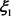，则

${\xi }_{1}={\xi }_{1}^{1}{r}_{1}\left(1,1\right)+{\xi }_{1}^{2}{r}_{2}\left(1,1\right)$ .

${|{\xi }_{1}|}^{2}=1$

${|{\xi }_{1}|}^{2}={\left({\xi }_{1}^{1}\right)}^{2}E\left(1,1\right)+2{\xi }^{1}{\xi }^{2}F\left(1,1\right)+{\left({\xi }_{1}^{2}\right)}^{2}G\left(1,1\right)=3{\left({\xi }_{1}^{1}\right)}^{2}+2{\xi }_{1}^{1}{\xi }_{1}^{2}+3{\left({\xi }_{1}^{2}\right)}^{2}=1$ ,

$3{\left({\xi }_{1}^{1}\right)}^{2}+2{\xi }_{1}^{1}{\xi }_{1}^{2}+3{\left({\xi }_{1}^{2}\right)}^{2}=1$ . (4.1)

${\xi }_{1}^{1}=-{\xi }_{1}^{2}$ (4.2)

$\left\{\begin{array}{l}{\xi }_{1}^{1}=\frac{1}{2}\\ {\xi }_{1}^{2}=-\frac{1}{2}\end{array}$$\left\{\begin{array}{l}{\xi }_{1}^{1}=-\frac{1}{2}\\ {\xi }_{1}^{2}=\frac{1}{2}\end{array}$ .

$\lambda =\frac{\sqrt{2}}{4}$ 对应的特征向量为

${\xi }_{1}={\xi }_{1}^{1}{r}_{u}\left(1,1\right)+{\xi }_{1}^{2}{r}_{v}\left(1,1\right)=\frac{1}{2}\left(1,1,1\right)\left(\begin{array}{c}i\\ j\\ k\end{array}\right)-\frac{1}{2}\left(1,-1,1\right)\left(\begin{array}{c}i\\ j\\ k\end{array}\right)=j$ ,

${\xi }_{1}={\xi }_{1}^{1}{r}_{u}\left(1,1\right)+{\xi }_{1}^{2}{r}_{v}\left(1,1\right)=-\frac{1}{2}\left(1,1,1\right)\left(\begin{array}{c}i\\ j\\ k\end{array}\right)+\frac{1}{2}\left(1,-1,1\right)\left(\begin{array}{c}i\\ j\\ k\end{array}\right)=-j$ .

$\left\{\begin{array}{l}{\xi }_{2}^{1}=\frac{\sqrt{2}}{4}\\ {\xi }_{2}^{2}=\frac{\sqrt{2}}{4}\end{array}$$\left\{\begin{array}{l}{\xi }_{2}^{1}=-\frac{\sqrt{2}}{4}\\ {\xi }_{2}^{2}=-\frac{\sqrt{2}}{4}\end{array}$ .

${\xi }_{2}={\xi }_{2}^{1}{r}_{u}\left(1,1\right)+{\xi }_{2}^{2}{r}_{v}\left(1,1\right)=\frac{\sqrt{2}}{4}\left(1,1,1\right)\left(\begin{array}{c}i\\ j\\ k\end{array}\right)+\frac{\sqrt{2}}{4}\left(1,-1,1\right)\left(\begin{array}{c}i\\ j\\ k\end{array}\right)=\frac{\sqrt{2}}{2}i+\frac{\sqrt{2}}{2}k$ ,

${\xi }_{2}={\xi }_{2}^{1}{r}_{u}\left(1,1\right)+{\xi }_{2}^{2}{r}_{v}\left(1,1\right)=-\frac{\sqrt{2}}{4}\left(1,1,1\right)\left(\begin{array}{c}i\\ j\\ k\end{array}\right)-\frac{\sqrt{2}}{4}\left(1,-1,1\right)\left(\begin{array}{c}i\\ j\\ k\end{array}\right)=-\frac{\sqrt{2}}{2}i-\frac{\sqrt{2}}{2}k$ .

Weingarten矩阵是一个2阶矩阵，求解2阶矩阵的特征值有一些规律，我们可以利用这样的规律使得计算更为简单。

$\omega =\left(\begin{array}{cc}\frac{\sqrt{2}}{16}& -\frac{3\sqrt{2}}{16}\\ -\frac{3\sqrt{2}}{16}& \frac{\sqrt{2}}{16}\end{array}\right)$

${\lambda }_{1}={a}_{11}+{a}_{12}=\frac{\sqrt{2}}{16}-\frac{3\sqrt{2}}{16}=-\frac{\sqrt{2}}{8}$ , 或 ${\lambda }_{2}={a}_{11}-{a}_{21}=\frac{\sqrt{2}}{16}-\left(-\frac{3\sqrt{2}}{16}\right)=\frac{\sqrt{2}}{4}$ .

${\lambda }^{2}-\frac{{M}^{2}}{EG}=0$ ,

${\lambda }_{1}=-\frac{M}{\sqrt{EG}}$${\lambda }_{2}=\frac{M}{\sqrt{EG}}$ .

$E\left(u,v\right)={r}_{u}\cdot {r}_{u}=1$ , $F\left(u,v\right)={r}_{u}\cdot {r}_{v}=0$ , $G\left(u,v\right)={u}^{2}+1$ ;

$L\left(u,v\right)={r}_{uu}\cdot n=0$ , $M\left(u,v\right)=r\left(u,v\right)\cdot n=-\frac{1}{\sqrt{{u}^{2}+1}}$ , $N\left(u,v\right)={r}_{vv}\cdot n=0$ .

2) 再求得Weingarten变换在原点 $\left(0,0,0\right)$ 的Weingarten矩阵。

$\left(x,y,z\right)=\left(0,0,0\right)$ 处所对应为

$\left\{\begin{array}{l}u\mathrm{cos}v=0\\ u\mathrm{sin}v=0\\ v=0\end{array}$ ,

$E\left(0,0\right)=1$ , $F\left(0,0\right)=1$ , $G\left(0,0\right)=1$ ,

$L\left(0,0\right)=N\left(0,0\right)=0$ , $M\left(0,0\right)=-1$ .

${\lambda }_{1}=-\frac{M}{\sqrt{EG}}=1$${\lambda }_{2}=\frac{M}{\sqrt{EG}}=-1$ .

$\lambda =1$ ，所对应的特征向量为 ${\xi }_{1}$ ，则

${\xi }_{1}={\xi }_{1}^{1}{r}_{1}\left(0,0\right)+{\xi }_{1}^{2}{r}_{2}\left(0,0\right)$ .

${|{\xi }_{1}|}^{2}=1$

${|{\xi }_{1}|}^{2}={\left({\xi }_{1}^{1}\right)}^{2}E\left(0,0\right)+2{\xi }_{1}^{1}{\xi }_{1}^{2}F\left(0,0\right)+{\left({\xi }_{1}^{2}\right)}^{2}G\left(0,0\right)={\left({\xi }_{1}^{1}\right)}^{2}+{\left({\xi }_{1}^{2}\right)}^{2}=1$ ,

${\left({\xi }_{1}^{1}\right)}^{2}+{\left({\xi }_{1}^{2}\right)}^{2}=1$ . (4.3)

$\left(\begin{array}{cc}0& -1\\ -1& 0\end{array}\right)\left(\begin{array}{c}{\xi }_{1}^{1}\\ {\xi }_{1}^{2}\end{array}\right)=\left(\begin{array}{c}{\xi }_{1}^{1}\\ {\xi }_{1}^{2}\end{array}\right)$ ,

${\xi }_{1}^{1}=-{\xi }_{1}^{2}$ . (4.4)

$\left\{\begin{array}{l}{\xi }_{1}^{1}=-\frac{\sqrt{2}}{2}\\ {\xi }_{1}^{2}=\frac{\sqrt{2}}{2}\end{array}$$\left\{\begin{array}{l}{\xi }_{1}^{1}=\frac{\sqrt{2}}{2}\\ {\xi }_{1}^{1}=-\frac{\sqrt{2}}{2}\end{array}$ .

$\lambda =1$ 对应的单位特征向量为

$\begin{array}{c}{\xi }_{1}={\xi }_{1}^{1}{r}_{1}\left(0,0\right)+{\xi }_{1}^{2}{r}_{2}\left(0,0\right)\\ =\frac{\sqrt{2}}{2}\left(0,0,0\right)\left(\begin{array}{c}i\\ j\\ k\end{array}\right)-\frac{\sqrt{2}}{2}\left(0,0,2\right)\left(\begin{array}{c}i\\ j\\ k\end{array}\right)\\ =-\sqrt{2}k\end{array}$

$\begin{array}{c}{\xi }_{1}={\xi }_{1}^{1}{r}_{1}\left(0,0\right)+{\xi }_{1}^{2}{r}_{2}\left(0,0\right)\\ =-\frac{\sqrt{2}}{2}\left(0,0,0\right)\left(\begin{array}{c}i\\ j\\ k\end{array}\right)+\frac{\sqrt{2}}{2}\left(0,0,2\right)\left(\begin{array}{c}i\\ j\\ k\end{array}\right)\\ =\sqrt{2}k\end{array}$

$\left\{\begin{array}{l}{\xi }_{2}^{1}=\frac{\sqrt{2}}{2}\\ {\xi }_{2}^{2}=\frac{\sqrt{2}}{2}\end{array}$$\left\{\begin{array}{l}{\xi }_{2}^{1}=-\frac{\sqrt{2}}{2}\\ {\xi }_{2}^{2}=-\frac{\sqrt{2}}{2}\end{array}$ .

$\begin{array}{c}{\xi }_{2}={\xi }_{2}^{1}{r}_{1}\left(0,0\right)+{\xi }_{2}^{2}{r}_{2}\left(0,0\right)\\ =\frac{\sqrt{2}}{2}\left(0,0,0\right)\left(\begin{array}{c}i\\ j\\ k\end{array}\right)+\frac{\sqrt{2}}{2}\left(0,0,2\right)\left(\begin{array}{c}i\\ j\\ k\end{array}\right)\\ =\sqrt{2}k\end{array}$

$\begin{array}{c}{\xi }_{2}={\xi }_{2}^{1}{r}_{1}\left(0,0\right)+{\xi }_{2}^{2}{r}_{2}\left(0,0\right)\\ =-\frac{\sqrt{2}}{2}\left(0,0,0\right)\left(\begin{array}{c}i\\ j\\ k\end{array}\right)-\frac{\sqrt{2}}{2}\left(0,0,2\right)\left(\begin{array}{c}i\\ j\\ k\end{array}\right)\\ =-\sqrt{2}k\end{array}$

$\omega =\left(\begin{array}{cc}\frac{L}{E}& 0\\ 0& \frac{N}{G}\end{array}\right)$ ,

$|\lambda I-\omega |=0$ ，得

$|\begin{array}{cc}\lambda -\frac{L}{E}& 0\\ 0& \lambda -\frac{N}{G}\end{array}|=0$ ,

${\lambda }_{1}=\frac{L}{E}$${\lambda }_{2}=\frac{N}{G}$ .

$\lambda =\frac{L}{E}$ 对应的特征向量为 ${\xi }_{1}$ ，则

$\omega \left(\begin{array}{c}{\xi }_{1}^{1}\\ {\xi }_{1}^{2}\end{array}\right)=\lambda \left(\begin{array}{c}{\xi }_{1}^{1}\\ {\xi }_{1}^{2}\end{array}\right)$ ,

$\left(\begin{array}{cc}\frac{L}{E}& 0\\ 0& \frac{N}{G}\end{array}\right)\left(\begin{array}{c}{\xi }_{1}^{1}\\ {\xi }_{1}^{2}\end{array}\right)=\frac{L}{E}\left(\begin{array}{c}{\xi }_{1}^{1}\\ {\xi }_{1}^{2}\end{array}\right)$ ,

$\frac{N}{G}=\frac{L}{E}$ ,

$r=\left(\left(R+r\mathrm{cos}u\right)\mathrm{cos}v,\left(R+r\mathrm{cos}u\right)\mathrm{sin}v,r\mathrm{sin}u\right)$ , $0\le u<2\text{π}$ , $0\le v<2\text{π}$

$E\left(u,v\right)={r}_{u}\cdot {r}_{u}={r}^{2}$ , $F\left(u,v\right)={r}_{u}\cdot {r}_{v}=0$ , $G\left(u,v\right)={r}_{v}\cdot {r}_{v}={\left(R+r\mathrm{cos}u\right)}^{2}$ ;

$L\left(u,v\right)={r}_{uu}\cdot n=r$ ,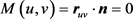, $N\left(u,v\right)={r}_{vv}\cdot n=\left(R+r\mathrm{cos}u\right)\mathrm{cos}u$ .

$\omega =\left(\begin{array}{cc}\frac{L}{E}& 0\\ 0& \frac{N}{G}\end{array}\right)=\left(\begin{array}{cc}\frac{1}{r}& 0\\ 0& \frac{\mathrm{cos}u}{R+r\mathrm{cos}u}\end{array}\right)$ ,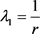${\lambda }_{2}=\frac{\mathrm{cos}u}{R+r\mathrm{cos}u}$ .

$\lambda =\frac{1}{r}$ 对应的特征向量为 ${\xi }_{1}$ ，由

$\left(\begin{array}{cc}\frac{1}{r}& 0\\ 0& \frac{\mathrm{cos}u}{R+r\mathrm{cos}u}\end{array}\right)\left(\begin{array}{c}{\xi }_{1}^{1}\\ {\xi }_{1}^{2}\end{array}\right)=\frac{1}{r}\left(\begin{array}{c}{\xi }_{1}^{1}\\ {\xi }_{1}^{2}\end{array}\right)$ ,

$\frac{\mathrm{cos}u}{R+r\mathrm{cos}u}=\frac{1}{r}$ ,

$\frac{1}{r}=\frac{\mathrm{cos}u}{R+r\mathrm{cos}u}$

5. 结束语

NOTES

*通讯作者。

 王萼芳, 石生明, 高等代数-3 [M], 北京: 高等教育出版社, 2003.

 徐克龙. 浅谈矩阵的特征向量特征值的意义[J]. 科技创新与应用, 2013(30): 297-297.

 王幼宁, 刘继, 微分几何讲义[M], 北京: 北京大学出版社, 2006: 113-116.

 吴春生. 浅议线性变换与矩阵的特征值与特征向量的关系[J]. 连云港师范高等专科学报, 2004(4): 75-76.

 梅向明, 黄敬之, 微分几何-2 [M], 北京: 高等教育出版社, 2004.

 智婕. 二阶矩阵特征值和特征向量的快速求法[J]. 洛阳师范学院学报, 2014(5): 5-7.

Top